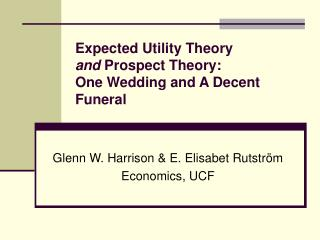# Expected Utility Theory and Prospect Theory: One Wedding and A Decent Funeral - PowerPoint PPT PresentationDownload PresentationExpected Utility Theory and Prospect Theory: One Wedding and A Decent Funeral

Expected Utility Theory and Prospect Theory: One Wedding and A Decent FuneralDownload Presentation## Expected Utility Theory and Prospect Theory: One Wedding and A Decent Funeral

- - - - - - - - - - - - - - - - - - - - - - - - - - - E N D - - - - - - - - - - - - - - - - - - - - - - - - - - -
##### Presentation Transcript

1. Expected Utility Theoryand Prospect Theory:One Wedding and A Decent Funeral Glenn W. Harrison & E. Elisabet Rutström Economics, UCF

2. Overview • Hypothesis tests assume just one data generating process • Whichever DGP explains more of the data is declared “the” DGP, and the others discarded • Consider lottery choice behavior • Assume EUT & Prospect Theory • Assume certain functional forms for the models generating the data • Allow for multiple DGP, united using mixture models and a grand likelihood function • Solve the model • Identify which subjects are better described by which DGP

3. Overview • Hypothesis tests assume just one data generating process • Whichever DGP explains more of the data is declared “the” DGP, and the others discarded • Consider lottery choice behavior: the Chapel in Vegas • Assume EUT & Prospect Theory: the Bride & Groom • Assume certain functional forms for the models generating the data: the Prenuptual Agreement • Allow for multiple DGP, united using mixture models and a grand likelihood function: the Wedding • Solve the model: Consummating the marriage • Identify which subjects are better described by which DGP: a Decent Funeral for the Representative Agent

4. Experimental Design • 158 UCF subjects make 60 lottery choices • Three selected at random and played out • Each subject received an initial endowment • Random endowment ~ [\$1, \$2, … \$10] • Three frames • Gain frame – prizes \$0, \$5, \$10 and \$15 [N=63] • Loss frame – endowment of \$15 and prizes -\$15, -\$10, -\$5 and \$0 [N=58] • Mixed frame – endowment of \$8 and prizes -\$8, -\$3, \$3 and \$8 [N=37] • Loss frames versus loss domains

5. Typical Screen Display

6. The Bride – EUT • Assume U(s,x) = (s+x)r • Assume probabilities for lottery as induced • EU = ∑k [pk x Uk] • Define latent index ∆EU = EUR - EUL • Define cumulative probability of observed choice by logistic G(∆EU) • Conditional log-likelihood of EUT then defined: ∑i [(lnG(∆EU)|yi=1)+(ln(1-G(∆EU))|yi=0)] • Need to estimate r

7. The Groom – PT • Assume U(x) = xá if x ≥ 0 • Assume U(x) = -λ(-x)â if x<0 • Assume w(p) = pγ/[ pγ + (1-p)γ ]1/γ • PU = ∑k [w(pk) x Uk] • Define latent index ∆PU = PUR - PUL • Define cumulative probability of observed choice by logistic G(∆PU) • Conditional log-likelihood of PT then defined: ∑i [(lnG(∆PU)|yi=1)+(ln(1-G(∆PU))|yi=0)] • Need to estimate á, â, λ and γ

8. The Nuptial • Grand-likelihood is just the probability weighted conditional likelihoods • Probability of EUT: πEUT • Probability of PT: πPT = 1-πEUT • Ln L(r, á, â, λ, γ, πEUT; y, X) = ∑i ln [(πEUT x LiEUT) +(πPT x LiPT)] • Need to jointly estimate r, á, â, λ, γ and πEUT • Two DGPs not nested, but could be • Easy to extend in principle to 3+ DGPs

9. Consummating the Marriage • Standard errors corrected for possible correlation of responses by same subject • The little pitter-patter of covariates: • X: {Female, Black, Hispanic, Age, Business, GPAlow} • Each parameter estimated as a linear function of X • Numerical issues

10. Result #1: Equal Billing for EUT & PT • Initially only assume heterogeneity of DGP • πEUT = 0.55 • So EUT wins by a (quantum) nose, but we do not declare winners that way • H0: πEUT = πPT = ½ has p-value of 0.49

11. Result #2: Estimates Are “Better” • When PT is assumed to characterize every data point, estimates are not so hot • Very little loss aversion • No probability weighting • But when estimated in mixture model, and only assumed to account for some of the choices, much more consistent with a priori beliefs

12. Result #3: Classifying Subjects • Now move to include covariates X • Subjects are either “clearly EUT” or “probably PT,” not two distinct modes

13. Conclusion • Sources of heterogeneity • Observable characteristics • Unobservable characteristics • Unobservable processes • Great potential to resolve some long-standing disputes • EUT versus PT • Exponential versus Hyperbolicky discounting • Calibrating for hypothetical bias in CVM • Serious technical issues to be addressed • Data needs – just increase N • Estimation problems